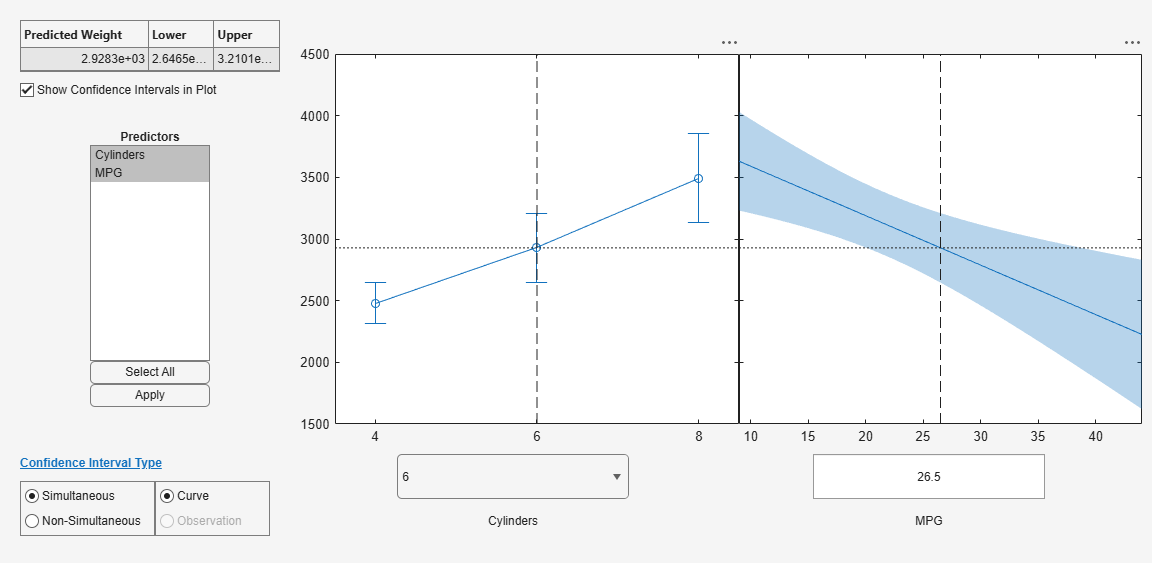# plotSlice

Plot of slices through fitted generalized linear regression surface

## Syntax

``plotSlice(mdl)``

## Description

example

````plotSlice(mdl)` creates a figure containing one or more plots, each representing a slice through the regression surface predicted by `mdl`. Each plot shows the fitted response values as a function of a single predictor variable, with the other predictor variables held constant.`plotSlice` also displays the 95% confidence bounds for the response values. Use the Bounds menu to choose the type of confidence bounds, and use the Predictors menu to select which predictors to use for each slice plot. For details, see Tips.```

## Examples

collapse all

Plot slices through a fitted generalized linear regression model surface.

Generate sample data using Poisson random numbers with two underlying predictors `X(:,1)` and `X(:,2)`.

```rng('default') % For reproducibility rndvars = randn(100,2); X = [2 + rndvars(:,1),rndvars(:,2)]; mu = exp(1 + X*[1;2]); y = poissrnd(mu);```

Create a generalized linear regression model of Poisson data.

`mdl = fitglm(X,y,'y ~ x1 + x2','Distribution','poisson');`

Create a slice plot.

`plotSlice(mdl)`The green line in each plot represents the predicted response values as a function of a single predictor variable, with the other predictor variables held constant. The red dotted lines are the 95% confidence bounds. The y-axis label includes the predicted response value and the corresponding confidence bound for the point selected by the vertical and horizontal lines. The x-axis label shows the predictor variable name and the predictor value for the selected point.

Move the vertical line in the `x1` plot to the right and observe the change in the y-axis label and the changes in the `x2` plot.## Input Arguments

collapse all

Generalized linear regression model, specified as a `GeneralizedLinearModel` object created using `fitglm` or `stepwiseglm`, or a `CompactGeneralizedLinearModel` object created using `compact`.

## Tips

• Use the Bounds menu in the figure window to choose the type of confidence bounds. You can choose Simultaneous or Non-Simultaneous. You can also choose No Bounds to have no confidence bounds.

• Simultaneous (default) — `plotSlice` computes confidence bounds for the curve of the response values using Scheffe's method. The range between the upper and lower confidence bounds contains the curve consisting of true response values with 95% confidence.

• Non-Simultaneous — `plotSlice` computes confidence bounds for the response value at each observation. The confidence interval for a response value at a specific predictor value contains the true response value with 95% confidence.

Simultaneous bounds are wider than separate bounds, because requiring the entire curve of response values to be within the bounds is stricter than requiring the response value at a single predictor value to be within the bounds.

• Use the Predictors menu in the figure window to select which predictors to use for each slice plot. If the regression model `mdl` includes more than eight predictors, `plotSlice` creates plots for the first five predictors by default.

## Alternative Functionality

• Use `predict` to return the predicted response values and confidence bounds. You can also specify the confidence level for confidence bounds by using the `'Alpha'` name-value pair argument of the `predict` function. Note that `predict` finds nonsimultaneous bounds by default, whereas `plotSlice` finds simultaneous bounds by default.

• A `GeneralizedLinearModel` object provides multiple plotting functions.

## Extended Capabilities

Introduced in R2012a